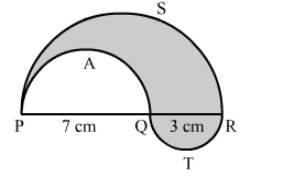# In the given figure, PSR, RTQ and PAQ are three semicircles of diameter 10 cm, 3 cm and 7 cm respectively.`
Question:

In the given figure, PSR, RTQ and PAQ are three semicircles of diameter 10 cm, 3 cm and 7 cm respectively. Find the perimeter of shaded region. [Use π= 3.14]Solution:

Perimeter of shaded region = Length of the arc PAQ + Length of the arc PSR + Length of the arc RTQ

$=\frac{1}{2} \times 2 \pi r_{1}+\frac{1}{2} \times 2 \pi r_{2}+\frac{1}{2} \times 2 \pi r_{3}$

$=\frac{1}{2} \times 2 \pi\left(\frac{7}{2}\right)+\frac{1}{2} \times 2 \pi\left(\frac{10}{2}\right)+\frac{1}{2} \times 2 \pi\left(\frac{3}{2}\right)$

$=\frac{7}{2} \pi+5 \pi+\frac{3}{2} \pi$

$=\frac{7}{2} \pi+\frac{3}{2} \pi+5 \pi$

$=5 \pi+5 \pi$

$=10 \pi$

$=31.4 \mathrm{~cm}$

Hence, the perimeter of shaded region is 31.4 cm.